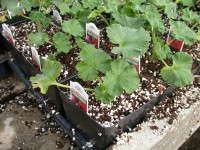# How PGRs Make You MoneyHow do you know when it is cost-effective to use plant growth regulators (PGRs)? Certainly they can be used to regulate plant growth and size, but that is just one part of the equation.

Chemical costs are the primary concern to growers. There is certainly a variable cost associated with using plant growth regulators. The growth controlled with PGRs allows for tighter plant spacing. Thus, there is less square foot per week cost, and a lower fixed cost assigned to the pot. So, we need to evaluate if the fixed cost savings is greater than the variable cost of the PGR application.

To provide an example, let’s use geraniums grown in a 4-inch square pot. This geranium crop will be grown for 10 weeks. For four weeks, plants will be placed pot tight at 4 inches by 4 inches. For the final six weeks, the plants will be spaced at 6 inches by 6 inches. Let’s calculate the amount of space the crop uses. This is how we evaluate the amount of fixed cost that will be charged to each individual pot. At 4 inches by 4 inches, the plant takes up one-third of a square foot times one-third of a square foot, or 0.1089 square feet. Multiply that by four weeks of growing time and that equals 0.436 square foot weeks. (See Figure 1 in slideshow below.)

At the 6-inch by 6-inch spacing, the plants take up half a square foot by half a square foot, so 0.5 x 0.5 = 0.25 square feet. Multiply that by six weeks of growing time and you have 1.5 square foot weeks. Adding those numbers together gives us 1.936 square foot weeks of growing time used for each individual pot.

Tighter Plant Spacings
So what difference does a PGR application make? We conducted an experiment in which Bonzi at 10 ppm was sprayed onto Medallion dark red geraniums. In this experiment, the resulting plants were 6.4 percent shorter and 16.7 percent smaller in diameter. When it comes to space allocation, we are interested in the change in plant diameter. Smaller-diameter plants can be spaced closer together.

So let’s calculate the improved space efficiency provided by the PGR application. It is impossible to improve the space utilization when the plants are spaced pot tight at 4 inches by 4 inches. Having smaller-sized plants will only affect the space utilized after the plants are spaced at 6 inches by 6 inches. So having 16.7 percent smaller plants means plants will only take up 83.4 percent of the space. We use that number and multiply it by the 1.5 square foot weeks. Thus, with the tighter pot spacing it will only take up 1.687 total square foot weeks to grow the crop.

Cost Comparison
Square foot per week cost normally varies with the size of the greenhouse. Ideally, you should know your own cost. For this example, we will use the cost of \$0.15, \$0.20 and \$0.25 respectively, which are representative of the large-, medium- and small-sized greenhouse.

Without a PGR application, a single pot occupies 1.936 square foot weeks of space. So the total fixed cost assigned to a single pot would be \$0.29, \$0.378 or \$0.489 respectively for a large, medium or small greenhouse. (See Figure 2 in slideshow below.)

With the tighter spacing possible with the PGR application, the fixed cost per pot is lower, at \$0.253, \$0.337 or \$0.422 respectively for the large-, medium- and small-sized greenhouse.

The difference is the savings offered by the tighter pot spacing when using a PGR. It equals 3.7 cents, 4.8 cents or 6.2 cents per pot respectively for the different-sized greenhouses.

Now, we calculate the cost of applying the PGR. When one calculates the chemical costs of spraying 10 ppm of Bonzi based on \$100 per quart, the cost to treat 9,000 pots would be less than \$5. The cost to treat an individual pot would be less than one-tenth of a cent. In this example, labor costs were not included since the time it takes to apply a foliar spray would be small.

The savings add up. Savings provided by the increased space efficiency would be \$37, \$48 or \$62 per 1,000 pots respectively for a large-, medium- or small-sized greenhouse.

In summary, there are economic benefits besides controlling excessive stretch when it comes to PGRs. PGRs allow for tighter pot spacing, which then translates into an increased profit per pot. Costs savings make the use of PGRs a key component when it comes to best managing practices for floriculture crops.